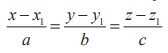Home | | Maths 12th Std | Condition for a line to lie in a plane

# Condition for a line to lie in a plane

We observe that a straight line will lie in a plane if every point on the line, lie in the plane and the normal to the plane is perpendicular to the line.

Condition for a line to lie in a plane

We observe that a straight line will lie in a plane if every point on the line, lie in the plane and the normal to the plane is perpendicular to the line.

i) If the linelies in the plane= d , then⋅= d and.= 0

ii) if the linelies in the plane Ax + By + Cz + D = 0 , then

Ax1 + By1 + Cz1 + D = 0 and aA + bB + cC = 0

### Example 6.45

Verify whether the linelies in the plane 5x y + z = 8 .

### Solution

Here, ( x1, y1, z1 ) = (3, 4, −3) and direction ratios of the given straight line are (a,b, c) = (−4, −7,12) . Direction ratios of the normal to the given plane are ( A, B,C ) = (5, −1,1) .

We observe that, the given point ( x1, y1, z1 ) = (3, 4, −3) satisfies the given plane 5x y + z = 8

Next, aA + bB + cC = (−4)(5) + (−7)(−1) + (12)(1) = −1 ≠ 0 . So, the normal to the plane is not perpendicular to the line. Hence, the given line does not lie in the plane.

Study Material, Lecturing Notes, Assignment, Reference, Wiki description explanation, brief detail
12th Mathematics : UNIT 6 : Applications of Vector Algebra : Condition for a line to lie in a plane |